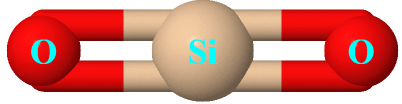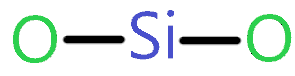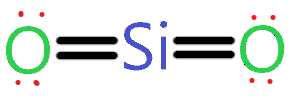# Silicon dioxide (SiO2) lewis dot structure, molecular geometry, polar or non-polar, hybridization

Home  > Chemistry Article > SiO2 lewis structure, molecular geometry, polar or non-polarSilicon dioxide is also known as silica made up of one silicon and two oxygen atoms with the chemical formula SiO2. It is mostly found in sand. Fine particles of silica found in quartz rock can cause long-term injury.

In this article, we will study Silicon dioxide (SiO2) lewis structure, molecular geometry, is it polar or non-polar, hybridization and bond angle, etc.

It can form crystals at room temperature like quartz. Silica particles can be suspended in the air and form non-explosive dust. Without wasting time let’s come to the topic just after covering its important properties.

Important properties of Silicon dioxide

• All forms of silica are odorless solids.
• It has the appearance of whitish powder.
• It is commonly found in nature as quartz.
• It is insoluble in water or poorly soluble in water.
• It has a molar mass of 60.08 g/mol.
• It has a boiling point of 2,950 °C and a melting point of 1,713 °C.
 Name of Molecule Silicon dioxide Chemical formula SiO2 Molecular geometry of SiO2 Linear Electron geometry of SiO2 Linear Hybridization Sp Bond angle 180º Total Valence electron for SiO2 16 The formal charge of SiO2 0
Page Contents

## How to draw Silicon dioxide (SiO2) lewis structure

SiO2 lewis’s structure contains two double bonds, a silicon atom at the central position, and two oxygen atoms surrounding it. There is no lone pair present on the central of SiO2 lewis structure but a total of 4 lone pairs are present on the outer atoms.

Let’s see how to draw SiO2 lewis dot structure step by step-

## Follow simple steps for drawing the SiO2 Lewis dot structure

### 1. Count total valence electron in SiO2

In the first step, we need to find how many valence electrons are available for SiO2 molecule. For this, you have two ways either know the periodic group of atoms or write the electronic configuration of individual atoms.

As silicon belongs to group 14th in the periodic table and oxygen belongs to the 16th group. So, the valence electron of silicon is 4 and for oxygen, it is 6.

⇒ Electronic configuration of oxygen = 1s2s2p4

⇒ Electronic configuration of silicon = 1s2 2s2 2p6 3s2 3p2

∴ Valence electron of silicon = 4

∴ Valence electron of oxygen = 6

∴ Total Valence electron available for drawing SiO2 lewis structure = 4 + 2*6 = 16 electrons### 2. Find the least electronegative atom and placed it at center

In this step, we will find the least electronegative atom in between silicon and oxygen for placing it at the center in the lewis diagram. As electronegativity increase from left to right in the periodic table, we got to know silicon is less electronegative than oxygen.

Therefore, place silicon at the center and oxygen spaced evenly around it.### 3. Connect silicon and oxygen with a single bond

Now in this step connect both oxygen atoms to the silicon atom with the help of a single bond for further drawing the SiO2 lewis structure.As one single bond contains two electrons and we used two single bonds in the above structure. It means we used 4 electrons from a total of 16 valence electrons.

∴ (16 -4) = 12 valence electrons

So, we are left with 12 valence electrons more.

### 4. Placed remaining valence electrons starting from outer atom first

In this step, we have to place the remaining valence electron around the outer atom (oxygen) first. We have 12 more valence electrons to work for and oxygen needs 8 electrons to achieve its octet. But oxygen already shares two electrons with the help of a single bond.

So, place 6 more electrons around each oxygen atom.As you see in the above structure, we have placed 6 electrons around each oxygen atom from the total of 12 remaining valence electrons. But this structure is not stable yet because silicon has only 4 electrons around it and it needs 4 more electrons to complete its octet.

Let’s complete it in the next step.

### 5. Complete central atom octet and make covalent bond if necessary

This is the final step for completing the SiO2 lewis diagram. As we know silicon needs 8 electrons to complete its octet but till now silicon has only 4 electrons.

For achieving the octet of silicon we will take the help from an oxygen atom lone pair electrons by converting it in form of a covalent bond.### Silicon dioxide (SiO2) lewis structure

As you see in the above SiO2 lewis dot structure, we convert 2 lone pairs of electrons of each oxygen atom to a covalent bond.

So, both atoms (silicon and oxygen) have 8 electrons in their valence shell. Hence we got our best and stable Silicon dioxide lewis structure.

## What is the electron and molecular geometry of SiO2(Silicon dioxide)?

The molecular geometry of SiO2 is very straightforward. Observe the lewis diagram of it and you got the answer. Yes, the molecular geometry of SiO2 is linear with Sp hybridization and a bond angle of 180º.

But why the molecular shape of SiO2 is linear? Read this article on CO2 molecular geometry you will get your answer briefly and in a simple explanation. Still, I will explain to you why the shape of SiO2 is linear.

Because there is no lone pair present on the central atom of the SiO2 lewis dot structure. So, there is no repulsion occur between bond pair and lone pair electrons around the central atom.

Also, a double bond between silicon and oxygen stabilizes the structure and Sp hybridization of the central atom also ensures that the molecular shape of SiO2 will be linear according to the VSEPR theory.### SiO2 molecular geometry

The electron geometry of SiO2 is also linear because no lone pair of electrons is present on the silicon. So, only a bond pair of electrons consider determining its electron geometry.

You can also find the electron and molecular geometry of SiO2 with the help of the VSEPR theory.

Let’s see how to do it.

## Follow some steps to find the molecular geometry of SiO2

### 1. Find hybridization of SiO2

For knowing what is the hybridization of SiO2? we have to find its hybridization number first. For this, we have a formula-

∴ H.N. = N.A. + L.P.

where H.N. = Hybridization number

⇒ N.A. = Number of atoms attached to the central atom

⇒ L.P. = lone pairs on that central atom

So, according to SiO2 lewis’s structure, silicon is the central atom that has no lone pair on it and two atoms attached to it.

∴ 2 + 0

H.N. = 2 is the hybridization number which means hybridization of SiO2 is Sp.

### 2. Find the Number of bond pairs and lone pairs present in the SiO2 lewis structure

As we already know, no lone pair of electrons is present on the central atom of the Silicon dioxide lewis structure. Also, two bond pairs are present, one each in between oxygen and silicon.

So, the number of lone pairs is zero and the bond pair is two.

Now according to the VSEPR theory, if any molecule has Sp hybridization and two bond pair with zero lone pairs present on the central atom then the molecular and electron geometry of that molecule is linear.

Let’s understand it with the help of the VSEPR chart.According to the above chart, A denoted the central atom, X denoted the bonded pair and N denoted the lone pairs of electrons. So, the Generic formula of SiO2 molecular geometry is AX2.

The bond angle of SiO2 is 180º because there is no lone pair present on the central atom that means there is no effect on the bond angle as there is no repulsion between lone and bond pair.

VSEPR theory says, “that the geometry around an atom that has only two bonds and no unshared electrons is a straight line”.## Silicon dioxide polarity: Is SiO2 polar or nonpolar?

Is SiO2 polar or non-polar? Clearly, SiO2 is non-polar because its net dipole moment is zero. Mostly molecular which have Sp hybridization and linear molecular geometry is non-polar in nature as they canceled the dipole moment generated along with the bond easily.

In the case of SiO2, the Si-O bond on both sides is arranged symmetrically which cancels out the dipole because the same element is present on both sides (left and right).

## Three factors that indicate the polarity of SiO2

### 1. Electronegativity:

Electronegativity directly influence the polarity of any atom or molecule. The larger the electronegativity difference between atoms higher is the polarity between those atoms.

The electronegativity of silicon is around 1.95 and for oxygen, it is 3.45. So, these electronegative differences between silicon and oxygen make the Si-O bond polar in nature. But the overall molecule is non-polar.

### 2. Dipole moment

The net dipole moment in SiO2 is zero because two dipole moment is generated from silicon to oxygen in the exact opposite direction to each other.

So, dipole cancels out each other as there is uniformly charge distributed all around and the structure is also symmetrical.

In mathematical terms, we can express dipole moment as D = Q×R

Dipole moment formula = charge on the atoms * the distance between them.

### 3. Geometrical or molecular shape:

We know the molecular shape of  SiO2 is linear which is highly symmetrical and the lewis structure of Silicon dioxide doesn’t have any lone pair of electrons so there is no distortion of shape happen which made it easy to cancel out the dipole moment generated along with the Si-O bond.

Hence all these factors show why SiO2 is a non-polar molecule.

## What is the formal charge in the SiO2 lewis dot structure and how to calculate it?

The formal charge is used to identify the most stable Lewis diagram. If the value of the formal charge is closest to zero then the lewis structure is most stable.

To calculate the formal charge, use the formula given below-

Formal charge = (Valence electrons – non-bonded electrons – 1/2 bonded electrons)

We will calculate the formal charge on the central atom of the SiO2 lewis diagram which is silicon.

⇒ Valence electron of Silicon = 4

⇒ Bonding electrons = 8

⇒ Non-bonding electrons(lone pairs electrons) = 0

Put these in given formal charge formula-

∴ (4 – 0 – 8/2)

= 0 is the formal charge on the Silicon atom.

## Uses of Silicon dioxide.

1. Silicon dioxide is used as a sedative.
2. It is used in pharmaceuticals.
3. It is used as a food additive.
4. It is used to remove the stickiness of powdered ingredients.
5. It is used as an anticaking agent.
6. It is used to produced concrete or cement.

## FAQ

### How many lone pair and bond pair electrons, Oxygen and Silicon have in the SiO2 lewis’s structure?

Each oxygen in the SiO2 lewis dot structure contains two lone pairs of electrons and 2 bonding pairs of electrons whereas silicon has zero lone pairs and 4 bonding pairs of electrons same as the CO2 lewis diagram.

### Why SiO2 is non-polar?

As we already discussed, SiO2 is non-polar because of three main reasons-

(a). It has Sp hybridization with a bond angle of 180º.

(b). The molecular geometry of SiO2 is linear and highly symmetrical therefore dipole moments can easily cancel out each other making it a non-polar molecule.

(c). There is no lone pair present on the central atom of the SiO2 lewis structure. So, no distortion happens in the structure and it remains symmetrical with uniformly charge distribution all around.

## Summary

• The net dipole moment of SiO2 is zero.
• The electron and molecular geometry of SiO2 are linear.
• The bond angle of Silicon dioxide is 180º and the hybridization of it is Sp.
• The total valence electron available for the Silicon dioxide lewis structure is 16.
• The formal charge in the SiO2 lewis dot structure is zero.
• SiO2 is a non-polar molecule.
##### Subscribe to Blog via Email

Join 2 other subscribers

Share it...